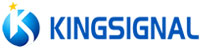language

#Stock Code:300252.SZ
English

## Vacuum Sealed Electrical Connector Compression Unit

author：kingsignal time：2017/08/14

The so-called "vacuum" refers to the gas in a given space, the pressure is less than 101325 pascal (that is, a standard atmospheric pressure of about 101KPa) gas state.

There are usually two ways to identify the degree of vacuum:

One is marked with "absolute pressure", "absolute vacuum" (ie, how much pressure is higher than "theoretical vacuum");

In the actual case, the absolute pressure of the vacuum seal connector is between 0 and 101.325 kPa. Absolute pressure values ??need to be measured with absolute pressure meters. At 20 ° C, altitude = 0, the initial value of the instrument (absolute vacuum gauge) used to measure the vacuum is 101.325KPa (ie, a standard atmospheric pressure).

The second is identified by "relative pressure", "relative vacuum" (ie, how much pressure is lower than "atmospheric pressure").

"Relative vacuum" refers to the difference between the pressure of the object to be measured and the atmospheric pressure at the measurement site. Measured with an ordinary vacuum gauge. In the absence of a vacuum (ie, atmospheric pressure), the initial value of the table is zero. When measuring a vacuum, its value is between 0 and -101.325KPa (usually expressed as a negative number).

For example, there is a vacuum sealed electrical connector measured value of -75KPa, then the vacuum electrical connector can be measured than the measured atmospheric pressure 75KPa vacuum state.

International vacuum industry common "vacuum", but also the most scientific is the absolute pressure identification; refers to the "extreme vacuum, absolute vacuum, absolute pressure", but "relative vacuum" (relative pressure, vacuum gauge pressure, Negative pressure) As the measurement method is simple, measuring instruments are very common, easy to buy and cheap, so it is also widely used. Theoretically the two can be converted each other, the two conversion method is as follows:

Relative Vacuum = Absolute Vacuum (Absolute Pressure) - Measure the air pressure at the location

For example: there is a miniature vacuum seal connector absolute pressure of 80KPa, then its relative vacuum is about 80-100 = -20Kpa, (measured at atmospheric pressure is assumed to be 100KPa) in the general vacuum table on the show -0.02MPa The

Commonly used vacuum units Pa, Kpa, Mpa, atmospheric pressure, kg (Kgf / cm2), mmHg, mbar, bar, PSI and so on. The approximate conversion relationship is as follows:

1MPa = 1000KPa

1KPa = 1000Pa

1 Atmospheric pressure = 100KPa = 0.1MPa

1 atm = 1 kg (Kgf / cm2) = 760 mmHg

1 Atmospheric pressure = 14.5PSI

1KPa = 10mbar

1bar = 1000mbar

Negative pressure out of the ventilation of the mine, the absolute pressure of the wind flow is less than the well outside the ball with the absolute high pressure, the relative pressure is negative, said negative pressure

Simply put, "negative pressure" is below the atmospheric pressure (that is, often say that an atmospheric pressure) gas pressure state. That is, we often say "vacuum". For example, when drinking a drink with a tube, the tube is negative; the inside of the sucker used to hang things, and the negative pressure.

The above is a brief description of the vacuum seal electrical connector pressure unit, learn more about the contents of the electrical connector, please pay attention to kingsignal official website.

BACK